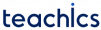# Half Subtractor

Half subtractor is a combinational circuit that performs subtraction of two bits. It accepts two binary digits as input and produces two binary digits, a difference and a borrow, in its output. The logic symbol of half subtractor is given below.

Expressions for Difference and Borrow can be derived from the following truth table and K-Map.

A B D Bout
0000
0111
1010
1100

Observe that the value of D is 1 when inputs A and B are at different logic levels. This can be expressed as XOR of two variables.Now the value of Bout is 1 only when input A is 0 and input B is 1. That is Bout can be expressed asFrom these equations, we can implement the logic circuit required as follows.

D is generated with an XOR gate with two inputs A and B, and the Bout is generated with an inverter and an AND gate.